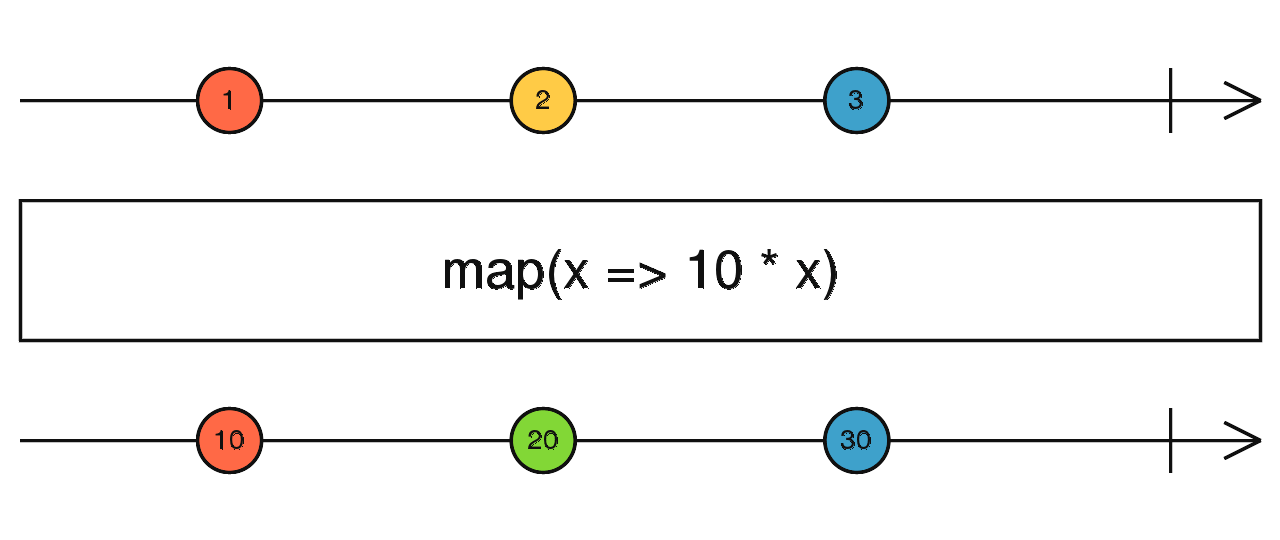/RxJS

# map

`function` `stable`

Applies a given `project` function to each value emitted by the source Observable, and emits the resulting values as an Observable.

### `map<T, R>(project: (value: T, index: number) => R, thisArg?: any): OperatorFunction<T, R>`

#### Parameters

 project The function to apply to each `value` emitted by the source Observable. The `index` parameter is the number `i` for the i-th emission that has happened since the subscription, starting from the number `0`. thisArg Optional. Default is `undefined`. An optional argument to define what `this` is in the `project` function.

#### Returns

`OperatorFunction<T, R>`: An Observable that emits the values from the source Observable transformed by the given `project` function.

## Description

Like Array.prototype.map(), it passes each source value through a transformation function to get corresponding output values.Similar to the well known `Array.prototype.map` function, this operator applies a projection to each value and emits that projection in the output Observable.

## Example

Map every click to the clientX position of that click

```import { fromEvent } from 'rxjs';
import { map } from 'rxjs/operators';

const clicks = fromEvent(document, 'click');
const positions = clicks.pipe(map(ev => ev.clientX));
positions.subscribe(x => console.log(x));```